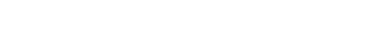# Sampling Validity

Reliability & Validity > Sampling Validity

## What is Sampling Validity?

Validity is how well a test measures what it is supposed to measure; Sampling validity (sometimes called logical validity) is how well the test covers all of the areas you want it to cover. For example, if you’re doing an assessment of effectiveness of math tutoring, you would want to include all areas of interest including: number comprehension, algebra skills and problem solving. An assessment would be a poor overall measure if it just tested algebra skills.

It’s usually not possible to cover every single area of interest from a single measure. Therefore, you’ll want to choose the most important items in your assessment. One way to do this is to consult with experts in the field to judge how well the items are representative of the content. In the above example, you could consult with mathematics educators.

A similar measure is item validity. While sampling validity is focused on the breadth of items, item validity focuses on their depth (Lodico & Spaulding, 2006).

## Alternate Definition

Not all authors define sampling variability in the same way. According to Krippendorff (2012), sampling variability refers to how well a population is accurately represented by a sample. It can be measured by the following formula:Where:

The right section of the equation is the sampling error formula (which can be called sampling invalidity), so the entire formula (1 – sampling error) is calculating sampling validity by subtracting the invalid portion of the test from 100%. Perhaps the most important aspect of the equation is that the larger the sample, the larger the sampling validity.

References:
Krippendorff, K. (2012). Content Analysis: An Introduction to its Methodology. SAGE, Apr. 12.
Lodico, M. & Spaulding, D. (2006). Methods in Educational Research: From Theory to Practice. John Wiley and Sons.

CITE THIS AS:
Stephanie Glen. "Sampling Validity" From StatisticsHowTo.com: Elementary Statistics for the rest of us! https://www.statisticshowto.com/sampling-validity/
---------------------------------------------------------------------------Need help with a homework or test question? With Chegg Study, you can get step-by-step solutions to your questions from an expert in the field. Your first 30 minutes with a Chegg tutor is free!